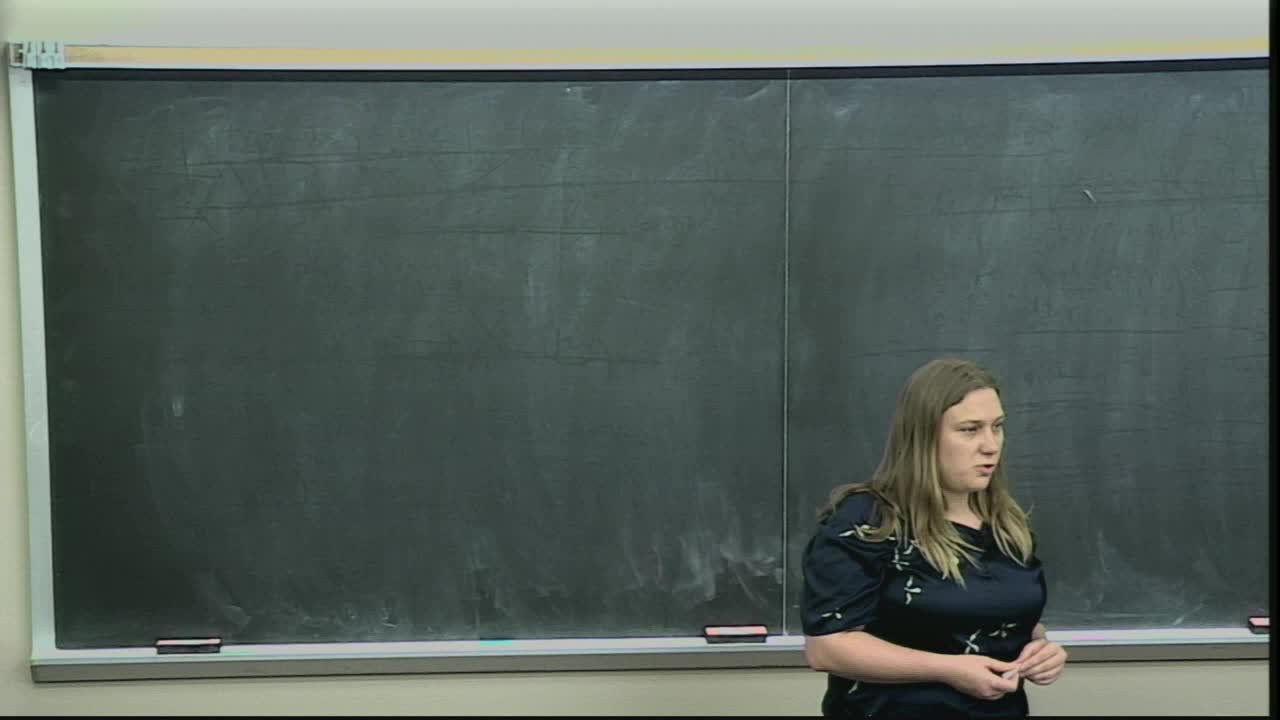## How many directions determine shape and other sufficiency results for topological transforms

May 21, 2018
IMA
Presenters: Katharine Turner

Watch Video

#### Abstract

The Persistent homology transform (PHT), the Euler Characteristic transform (ECT) and their variants are useful for statistical shape analysis. The idea is that a topological summary of an embedded shape is calculated for height functions corresponding for the different directions in the sphere, capturing the topology from the perspective of each of those directions. Euler calculus provides an simple yet very general proof that the Euler curves/persistence diagrams from all the directions complete describe the any compact o-minimal. The point of this talk is to investigate the structure of these transforms in order to prove other sufficiency results. We know that o-minimal sets can naturally be stratified into manifold pieces. This is important because it implies that the constructions that underly the ECT and PHT are also stratified. The upshot of this observation is that the ECT of a particular shape M should be determined, in some sense, by finitely many directions. We can describe this stratification explicitly in the case of finite simplicial complexes and provide an algorithmic approach to computing the PHT/ECT from a bounded number of directions. We also use the piecewise linear nature of the stratified map to prove that the distribution of the topological transforms around the sphere is sufficient to determine a finite simplicial complex up to alignment. This is important as alignment in statistical shape analysis is considered a hard problem.